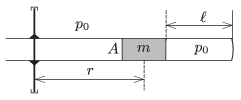Mathematical and Physical Journal
for High Schools
Issued by the MATFUND Foundation
 Already signed up? New to KöMaL?

#Problem P. 4811. (February 2016)

P. 4811. A piston of mass $\displaystyle m=2~{\rm kg}$ confines an air column of height $\displaystyle \ell=30~{\rm cm}$ in a cylinder of cross section $\displaystyle A=10~{\rm cm}^2$. Both values of the internal and the external pressure are $\displaystyle p_0=10^5$ Pa. The cylinder can be rotated about a vertical axle. In the stationary position the middle of the piston is at a distance of $\displaystyle r=0.5$ m from the axle.$\displaystyle a)$ By what factor will the density of the gas increase if the cylinder is rotated with an angular speed of $\displaystyle \omega=3~{\rm s}^{-1}$.

$\displaystyle b)$ During the rotation, to what value should the external pressure be changed in order that the piston moves back to its original position? The temperature remains constant all the time.

(4 pont)

Deadline expired on March 10, 2016.

### Statistics:

 71 students sent a solution. 4 points: 53 students. 3 points: 10 students. 2 points: 5 students. 1 point: 2 students. 0 point: 1 student.

Problems in Physics of KöMaL, February 2016